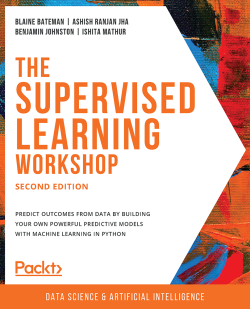# The Supervised Learning Workshop - Second Edition3 (1 reviews total)
By Blaine Bateman , Ashish Ranjan Jha , Benjamin Johnston and 1 more
• \$25.99 eBook
• \$36.99 Print + eBook
What do you get with a Packt Subscription?

• Constantly updated with 100+ new titles each month
• Breadth and depth in over 1,000+ technologies

Would you like to understand how and why machine learning techniques and data analytics are spearheading enterprises globally? From analyzing bioinformatics to predicting climate change, machine learning plays an increasingly pivotal role in our society.

Although the real-world applications may seem complex, this book simplifies supervised learning for beginners with a step-by-step interactive approach. Working with real-time datasets, you’ll learn how supervised learning, when used with Python, can produce efficient predictive models.

Starting with the fundamentals of supervised learning, you’ll quickly move to understand how to automate manual tasks and the process of assessing date using Jupyter and Python libraries like pandas. Next, you’ll use data exploration and visualization techniques to develop powerful supervised learning models, before understanding how to distinguish variables and represent their relationships using scatter plots, heatmaps, and box plots. After using regression and classification models on real-time datasets to predict future outcomes, you’ll grasp advanced ensemble techniques such as boosting and random forests. Finally, you’ll learn the importance of model evaluation in supervised learning and study metrics to evaluate regression and classification tasks.

By the end of this book, you’ll have the skills you need to work on your real-life supervised learning Python projects.

Publication date:
February 2020
Publisher
Packt
Pages
532
ISBN
9781800209046

# 1. Fundamentals

Overview

This chapter introduces you to supervised learning, using Anaconda to manage coding environments, and using Jupyter notebooks to create, manage, and run code. It also covers some of the most common Python packages used in supervised learning: pandas, NumPy, Matplotlib, and seaborn. By the end of this chapter, you will be able to install and load Python libraries into your development environment for use in analysis and machine learning problems. You will also be able to load an external data source using pandas, and use a variety of methods to search, filter, and compute descriptive statistics of the data. This chapter will enable you to gauge the potential impact of various issues such as missing data, class imbalance, and low sample size within the data source.

# Introduction

The study and application of machine learning and artificial intelligence has recently been the source of much interest and research in the technology and business communities. Advanced data analytics and machine learning techniques have shown great promise in advancing many sectors, such as personalized healthcare and self-driving cars, as well as in solving some of the world's greatest challenges, such as combating climate change (see Tackling Climate Change with Machine Learning: https://arxiv.org/pdf/1906.05433.pdf).

This book has been designed to help you to take advantage of the unique confluence of events in the field of data science and machine learning today. Across the globe, private enterprises and governments are realizing the value and efficiency of data-driven products and services. At the same time, reduced hardware costs and open source software solutions are significantly reducing the barriers to entry of learning and applying machine learning techniques.

Here, we will focus on supervised machine learning (or, supervised learning for short). We'll explain the different types of machine learning shortly, but let's begin with some quick information. The now-classic example of supervised learning is developing an algorithm to distinguish between pictures of cats and dogs. The supervised part arises from two aspects; first, we have a set of pictures where we know the correct answers. We call such data labeled data. Second, we carry out a process where we iteratively test our algorithm's ability to predict "cat" or "dog" given pictures, and we make corrections to the algorithm when the predictions are incorrect. This process, at a high level, is similar to teaching children. However, it generally takes a lot more data to train an algorithm than to teach a child to recognize cats and dogs! Fortunately, there are rapidly growing sources of data at our disposal. Note the use of the words learning and train in the context of developing our algorithm. These might seem to be giving human qualities to our machines and computer programs, but they are already deeply ingrained in the machine learning (and artificial intelligence) literature, so let's use them and understand them. Training in our context here always refers to the process of providing labeled data to an algorithm and making adjustments to the algorithm to best predict the labels given the data. Supervised means that the labels for the data are provided within the training, allowing the model to learn from these labels.

Let's now understand the distinction between supervised learning and other forms of machine learning.

## When to Use Supervised Learning

Generally, if you are trying to automate or replicate an existing process, the problem is a supervised learning problem. As an example, let's say you are the publisher of a magazine that reviews and ranks hairstyles from various time periods. Your readers frequently send you far more images of their favorite hairstyles for review than you can manually process. To save some time, you would like to automate the sorting of the hairstyle images you receive based on time periods, starting with hairstyles from the 1960s and 1980s, as you can see in the following figure:Figure 1.1: Images of hairstyles from different time periods

To create your hairstyles-sorting algorithm, you start by collecting a large sample of hairstyle images and manually labeling each one with its corresponding time period. Such a dataset (known as a labeled dataset) is the input data (hairstyle images) for which the desired output information (time period) is known and recorded. This type of problem is a classic supervised learning problem; we are trying to develop an algorithm that takes a set of inputs and learns to return the answers that we have told it are correct.

## Python Packages and Modules

Python is one of the most popular programming languages used for machine learning, and is the language used here.

While the standard features that are included in Python are certainly feature-rich, the true power of Python lies in the additional libraries (also known as packages), which, thanks to open source licensing, can be easily downloaded and installed through a few simple commands. In this book, we generally assume your system has been configured using Anaconda, which is an open source environment manager for Python. Depending on your system, you can configure multiple virtual environments using Anaconda, each one configured with specific packages and even different versions of Python. Using Anaconda takes care of many of the requirements to get ready to perform machine learning, as many of the most common packages come pre-built within Anaconda. Refer to the preface for Anaconda installation instructions.

In this book, we will be using the following additional Python packages:

• NumPy (pronounced Num Pie and available at https://www.numpy.org/): NumPy (short for numerical Python) is one of the core components of scientific computing in Python. NumPy provides the foundational data types from which a number of other data structures derive, including linear algebra, vectors and matrices, and key random number functionality.
• SciPy (pronounced Sigh Pie and available at https://www.scipy.org): SciPy, along with NumPy, is a core scientific computing package. SciPy provides a number of statistical tools, signal processing tools, and other functionality, such as Fourier transforms.
• pandas (available at https://pandas.pydata.org/): pandas is a high-performance library for loading, cleaning, analyzing, and manipulating data structures.
• Matplotlib (available at https://matplotlib.org/): Matplotlib is the foundational Python library for creating graphs and plots of datasets and is also the base package from which other Python plotting libraries derive. The Matplotlib API has been designed in alignment with the Matlab plotting library to facilitate an easy transition to Python.
• Seaborn (available at https://seaborn.pydata.org/): Seaborn is a plotting library built on top of Matplotlib, providing attractive color and line styles as well as a number of common plotting templates.
• Scikit-learn (available at https://scikit-learn.org/stable/): Scikit-learn is a Python machine learning library that provides a number of data mining, modeling, and analysis techniques in a simple API. Scikit-learn includes a number of machine learning algorithms out of the box, including classification, regression, and clustering techniques.

These packages form the foundation of a versatile machine learning development environment, with each package contributing a key set of functionalities. As discussed, by using Anaconda, you will already have all of the required packages installed and ready for use. If you require a package that is not included in the Anaconda installation, it can be installed by simply entering and executing the following code in a Jupyter notebook cell:

`!conda install <package name>`

As an example, if we wanted to install Seaborn, we'd run the following command:

`!conda install seaborn`

To use one of these packages in a notebook, all we need to do is import it:

`import matplotlib`

`pandas` has the ability to read and write a number of different file formats and data structures, including CSV, JSON, and HDF5 files, as well as SQL and Python Pickle formats. The pandas input/output documentation can be found at https://pandas.pydata.org/pandas-docs/stable/user_guide/io.html. We will continue to look into the `pandas` functionality by loading data via a CSV file.

Note

The dataset used in this chapter is available on our GitHub repository via the following link: https://packt.live/2vjyPK9. Once you download the entire repository on your system, you can find the dataset in the `Datasets` folder. Furthermore, this dataset is the Titanic: Machine Learning from Disaster dataset, which was originally made available at https://www.kaggle.com/c/Titanic/data.

The dataset contains a roll of the guests on board the famous ship Titanic, as well as their age, survival status, and number of siblings/parents. Before we get started with loading the data into Python, it is critical that we spend some time looking over the information provided for the dataset so that we can have a thorough understanding of what it contains. Download the dataset and place it in the directory you're working in.

Looking at the description for the data, we can see that we have the following fields available:

• `survival`: This tells us whether a given person survived (`0` = No, `1` = Yes).
• `pclass`: This is a proxy for socio-economic status, where first class is upper, second class is middle, and third class is lower status.
• `sex`: This tells us whether a given person is male or female.
• `age`: This is a fractional value if less than 1; for example, 0.25 is 3 months. If the age is estimated, it is in the form of xx.5.
• `sibsp`: A sibling is defined as a brother, sister, stepbrother, or stepsister, and a spouse is a husband or wife.
• `parch`: A parent is a mother or father, while a child is a daughter, son, stepdaughter, or stepson. Children that traveled only with a nanny did not travel with a parent. Thus, 0 was assigned for this field.
• `ticket`: This gives the person's ticket number.
• `fare`: This is the passenger's fare.
• `cabin`: This tells us the passenger's cabin number.
• `embarked`: The point of embarkation is the location where the passenger boarded the ship.

Note that the information provided with the dataset does not give any context as to how the data was collected. The `survival`, `pclass`, and `embarked` fields are known as categorical variables as they are assigned to one of a fixed number of labels or categories to indicate some other information. For example, in `embarked`, the `C` label indicates that the passenger boarded the ship at Cherbourg, and the value of `1` in `survival` indicates they survived the sinking.

In this exercise, we will read our Titanic dataset into Python and perform a few basic summary operations on it:

1. Open a new Jupyter notebook.
2. Import the `pandas` and `numpy` packages using shorthand notation:
```import pandas as pd
import numpy as np```
3. Open the `titanic.csv` file by clicking on it in the Jupyter notebook home page as shown in the following figure: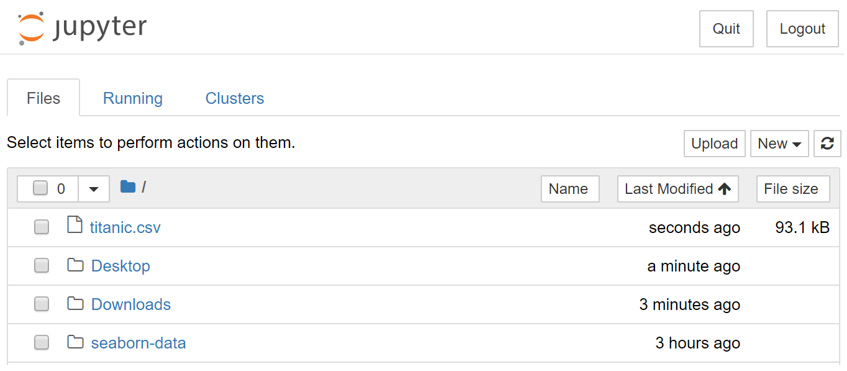Figure 1.2: Opening the CSV file

The file is a CSV file, which can be thought of as a table, where each line is a row in the table and each comma separates columns in the table. Thankfully, we don't need to work with these tables in raw text form and can load them using `pandas`: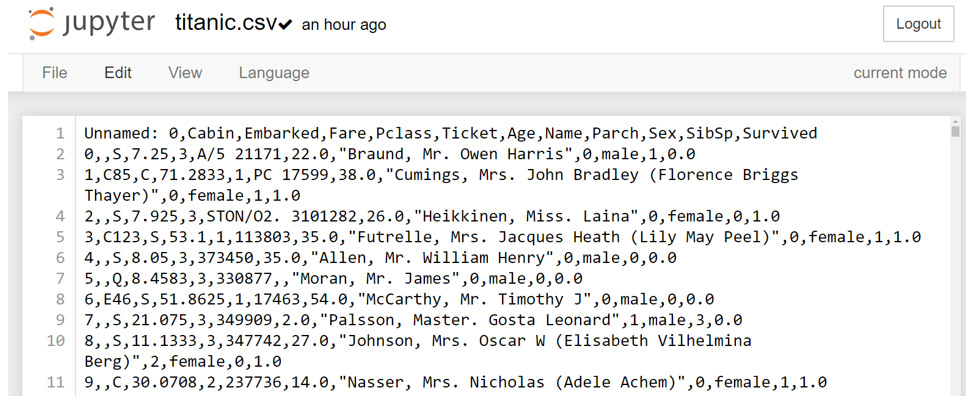Figure 1.3: Contents of the CSV file

Note

Take a moment to look up the pandas documentation for the `read_csv` function at https://pandas.pydata.org/pandas-docs/stable/reference/api/pandas.read_csv.html. Note the number of different options available for loading CSV data into a pandas DataFrame.

4. In an executable Jupyter notebook cell, execute the following code to load the data from the file:
`df = pd.read_csv(r'..\Datasets\titanic.csv')`

The pandas DataFrame class provides a comprehensive set of attributes and methods that can be executed on its own contents, ranging from sorting, filtering, and grouping methods to descriptive statistics, as well as plotting and conversion.

Note

Open and read the documentation for pandas DataFrame objects at https://pandas.pydata.org/pandas-docs/stable/reference/frame.html.

5. Read the first ten rows of data using the `head()` method of the DataFrame:

Note

The `#` symbol in the code snippet below denotes a code comment. Comments are added into code to help explain specific bits of logic.

`df.head(10) # Examine the first 10 samples`

The output will be as follows: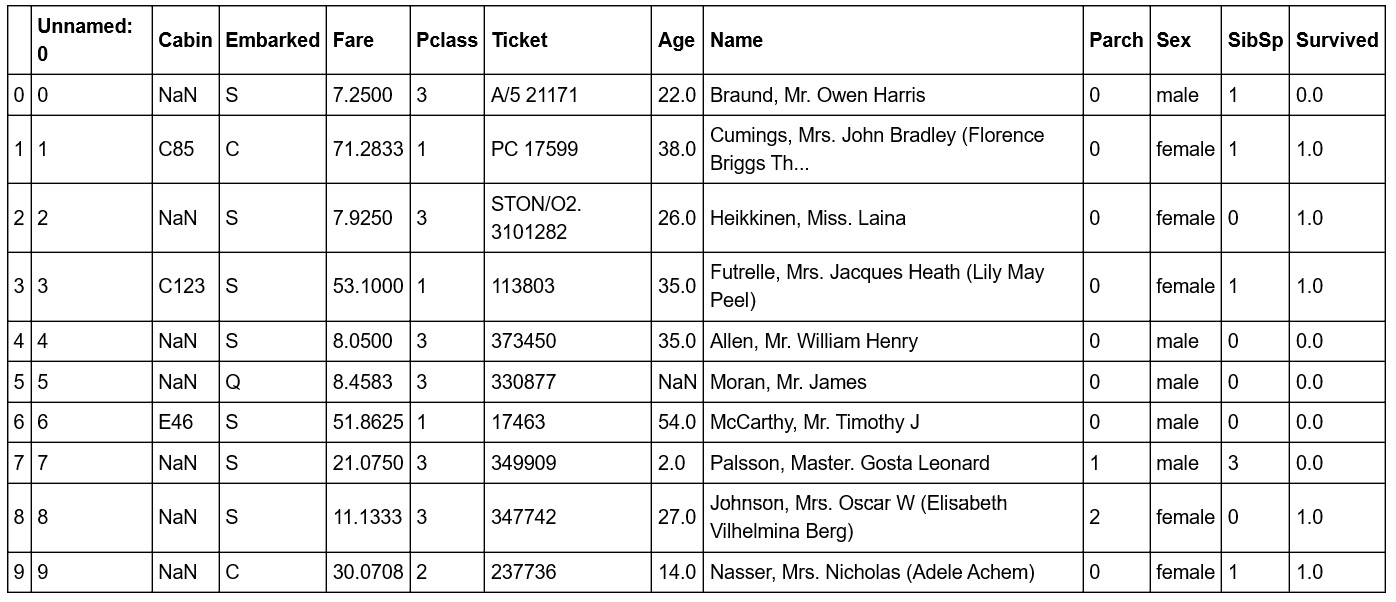Figure 1.4: Reading the first 10 rows

Note

To access the source code for this specific section, please refer to https://packt.live/2Ynb7sf.

You can also run this example online at https://packt.live/2BvTRrG. You must execute the entire Notebook in order to get the desired result.

In this sample, we have a visual representation of the information in the DataFrame. We can see that the data is organized in a tabular, almost spreadsheet-like structure. The different types of data are organized into columns, while each sample is organized into rows. Each row is assigned an index value and is shown as the numbers 0 to 9 in bold on the left-hand side of the DataFrame. Each column is assigned to a label or name, as shown in bold at the top of the DataFrame.

The idea of a DataFrame as a kind of spreadsheet is a reasonable analogy. As we will see in this chapter, we can sort, filter, and perform computations on the data just as you would in a spreadsheet program. While it's not covered in this chapter, it is interesting to note that DataFrames also contain pivot table functionality, just like a spreadsheet (https://pandas.pydata.org/pandas-docs/stable/reference/api/pandas.pivot_table.html).

## Exercise 1.02: Indexing and Selecting Data

Now that we have loaded some data, let's use the selection and indexing methods of the DataFrame to access some data of interest. This exercise is a continuation of Exercise 1.01, Loading and Summarizing the Titanic Dataset:

1. Select individual columns in a similar way to a regular dictionary by using the labels of the columns, as shown here:
`df['Age']`

The output will be as follows:

```0       22.0
1       38.0
2       26.0
3       35.0
4       35.0
...
1304     NaN
1305    39.0
1306    38.5
1307     NaN
1308     NaN
Name: Age, Length: 1309, dtype: float64```

If there are no spaces in the column name, we can also use the dot operator. If there are spaces in the column names, we will need to use the bracket notation:

`df.Age`

The output will be as follows:

```0       22.0
1       38.0
2       26.0
3       35.0
4       35.0
...
1304     NaN
1305    39.0
1306    38.5
1307     NaN
1308     NaN
Name: Age, Length: 1309, dtype: float64```
2. Select multiple columns at once using bracket notation, as shown here:
`df[['Name', 'Parch', 'Sex']]`

The output will be as follows: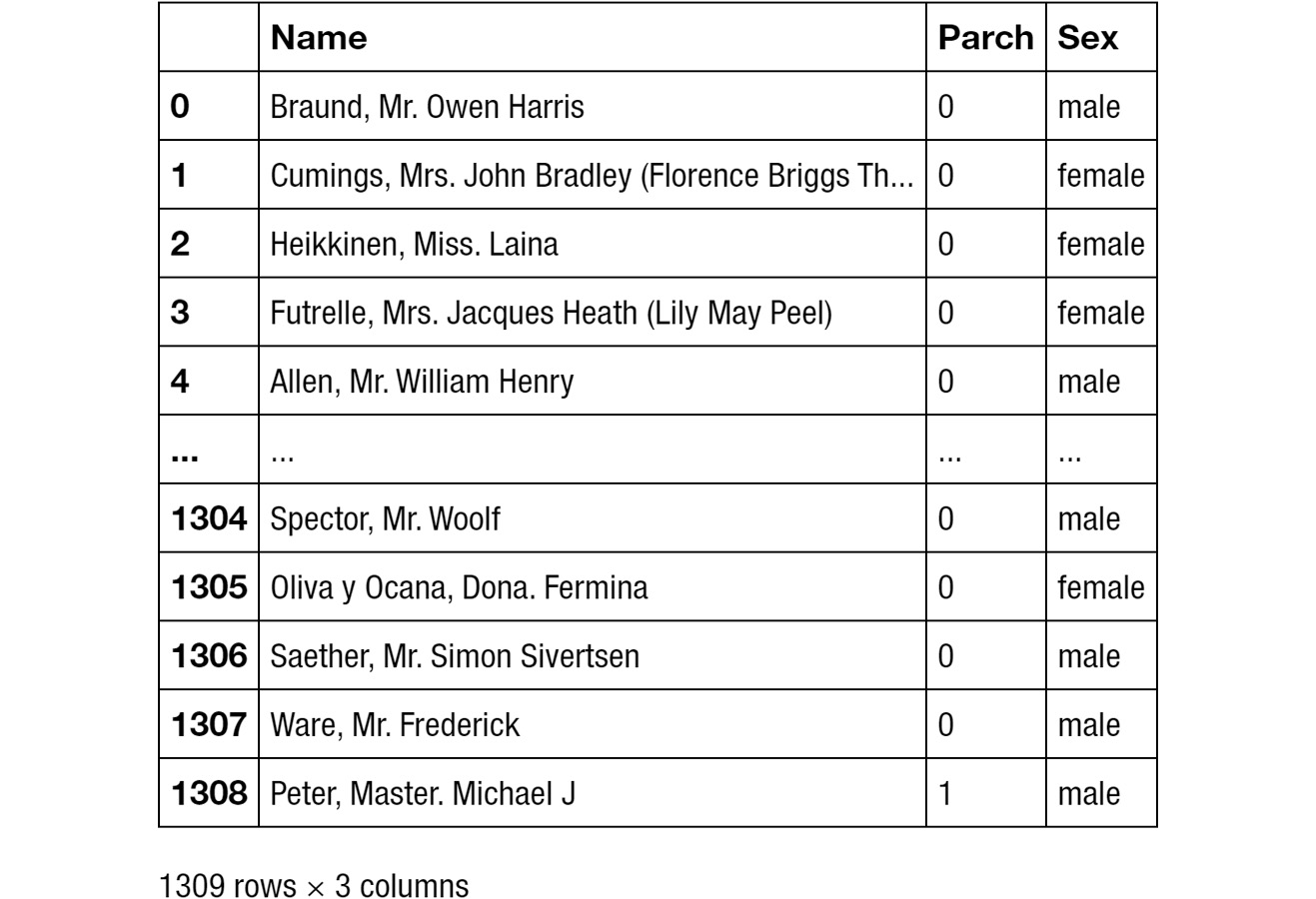Figure 1.5: Selecting multiple columns

Note

The output has been truncated for presentation purposes.

3. Select the first row using `iloc`:
`df.iloc`

The output will be as follows: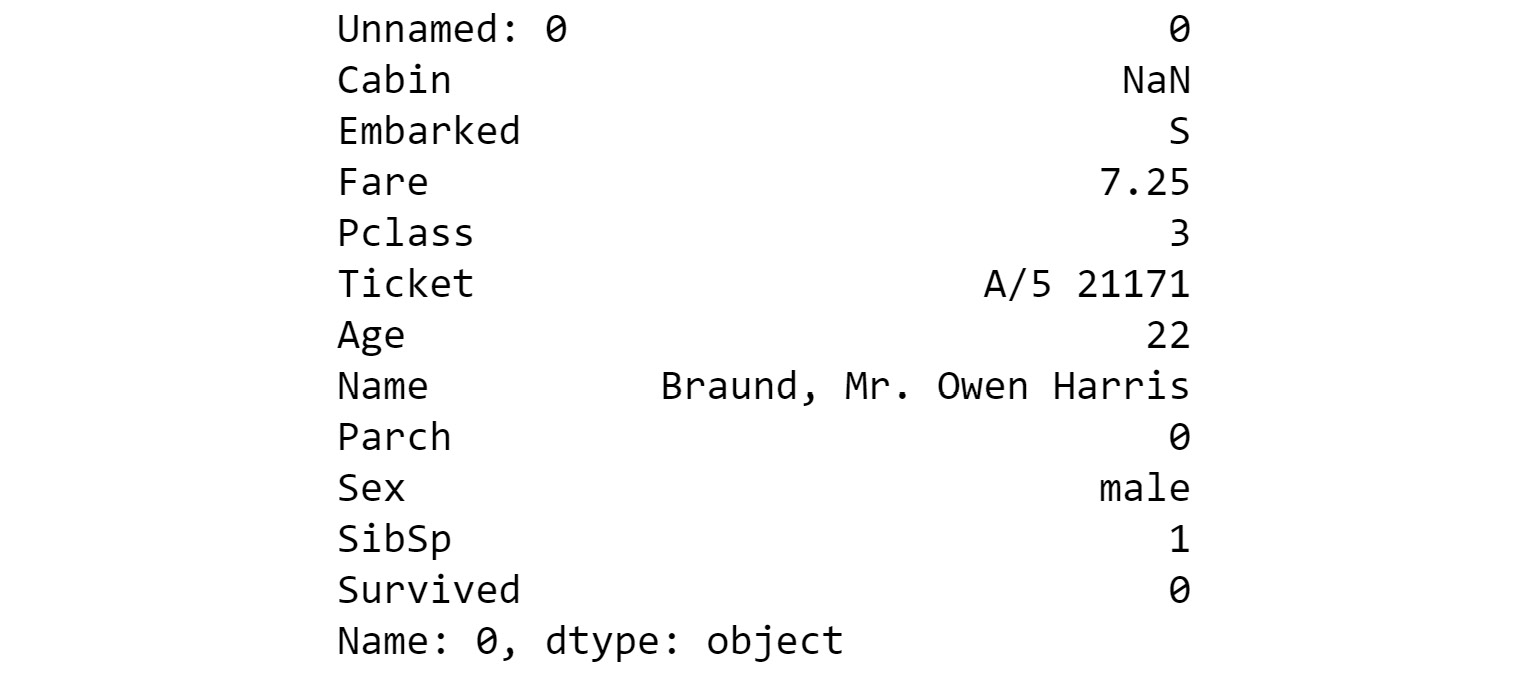Figure 1.6: Selecting the first row

4. Select the first three rows using `iloc`:
`df.iloc[[0,1,2]]`

The output will be as follows: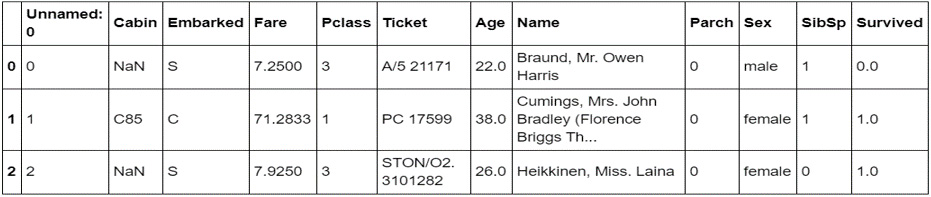Figure 1.7: Selecting the first three rows

5. Next, get a list of all of the available columns:
```columns = df.columns # Extract the list of columns
print(columns)```

The output will be as follows: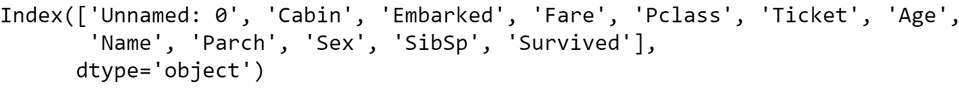Figure 1.8: Getting all the columns

6. Use this list of columns and the standard Python slicing syntax to get columns 2, 3, and 4, and their corresponding values:
`df[columns[1:4]] # Columns 2, 3, 4`

The output will be as follows: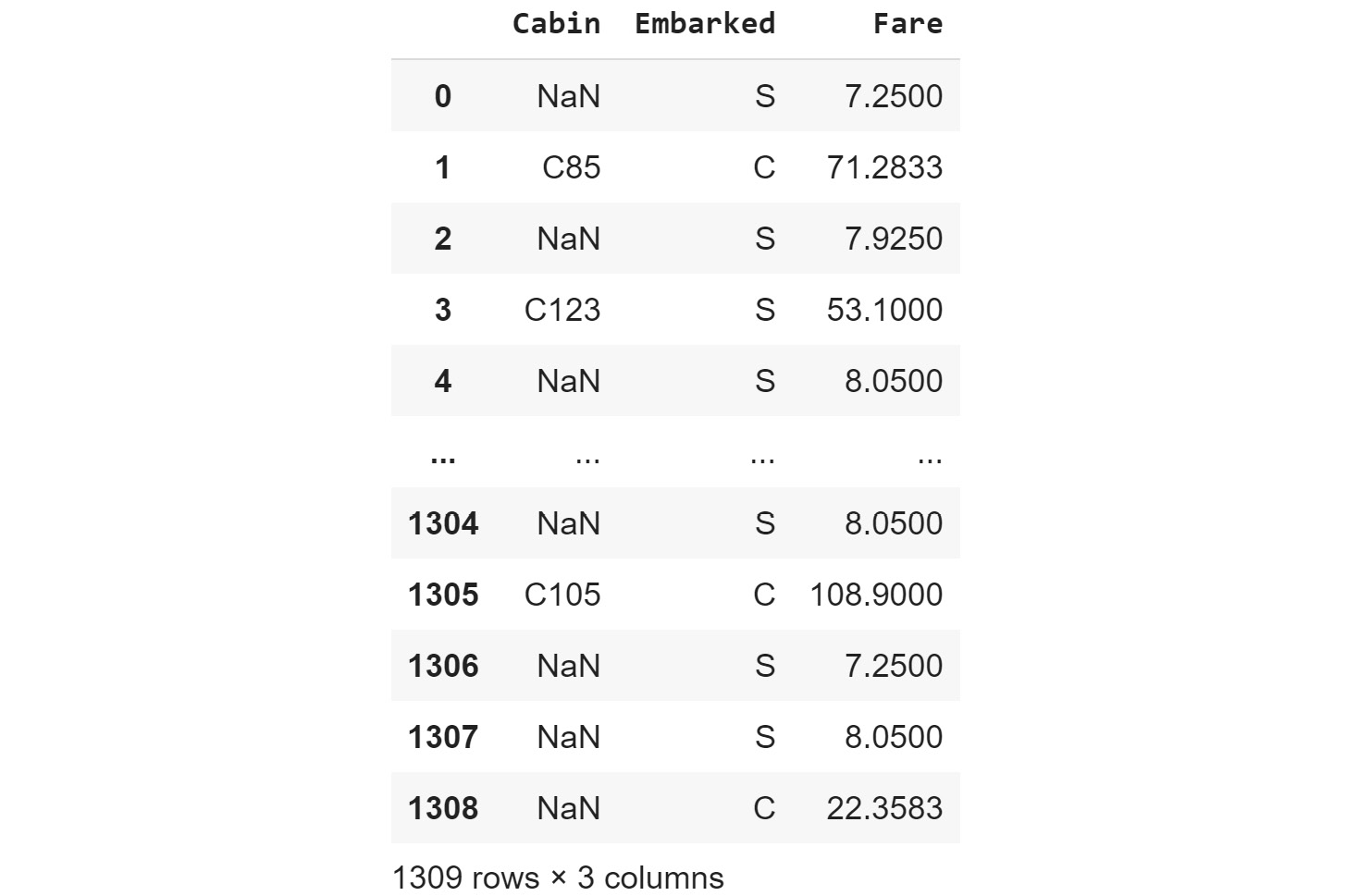Figure 1.9: Getting the second, third, and fourth columns

7. Use the `len` operator to get the number of rows in the DataFrame:
`len(df)`

The output will be as follows:

`1309`
8. Get the value for the `Fare` column in row 2 using the row-centric method:
`df.iloc['Fare'] # Row centric`

The output will be as follows:

`7.925`
9. Use the dot operator for the column, as follows:
`df.iloc.Fare # Row centric`

The output will be as follows:

`7.925`
10. Use the column-centric method, as follows:
`df['Fare'] # Column centric`

The output will be as follows:

`7.925`
11. Use the column-centric method with the dot operator, as follows:
`df.Fare # Column centric`

The output will be as follows:

`7.925`

Note

To access the source code for this specific section, please refer to https://packt.live/2YmA7jb.

You can also run this example online at https://packt.live/3dmk0qf. You must execute the entire Notebook in order to get the desired result.

In this exercise, we have seen how to use pandas' `read_csv()` function to load data into Python within a Jupyter notebook. We then explored a number of ways that pandas, by presenting the data in a DataFrame, facilitates selecting specific items in a DataFrame and viewing the contents. With these basics understood, let's look at some more advanced ways to index and select data.

## Exercise 1.03: Advanced Indexing and Selection

With the basics of indexing and selection under our belt, we can turn our attention to more advanced indexing and selection. In this exercise, we will look at a few important methods for performing advanced indexing and selecting data. This exercise is a continuation of Exercise 1.01, Loading and Summarizing the Titanic Dataset:

1. Create a list of the passengers' names and ages for those passengers under the age of 21, as shown here:
```child_passengers = df[df.Age  < 21][['Name', 'Age']]

The output will be as follows: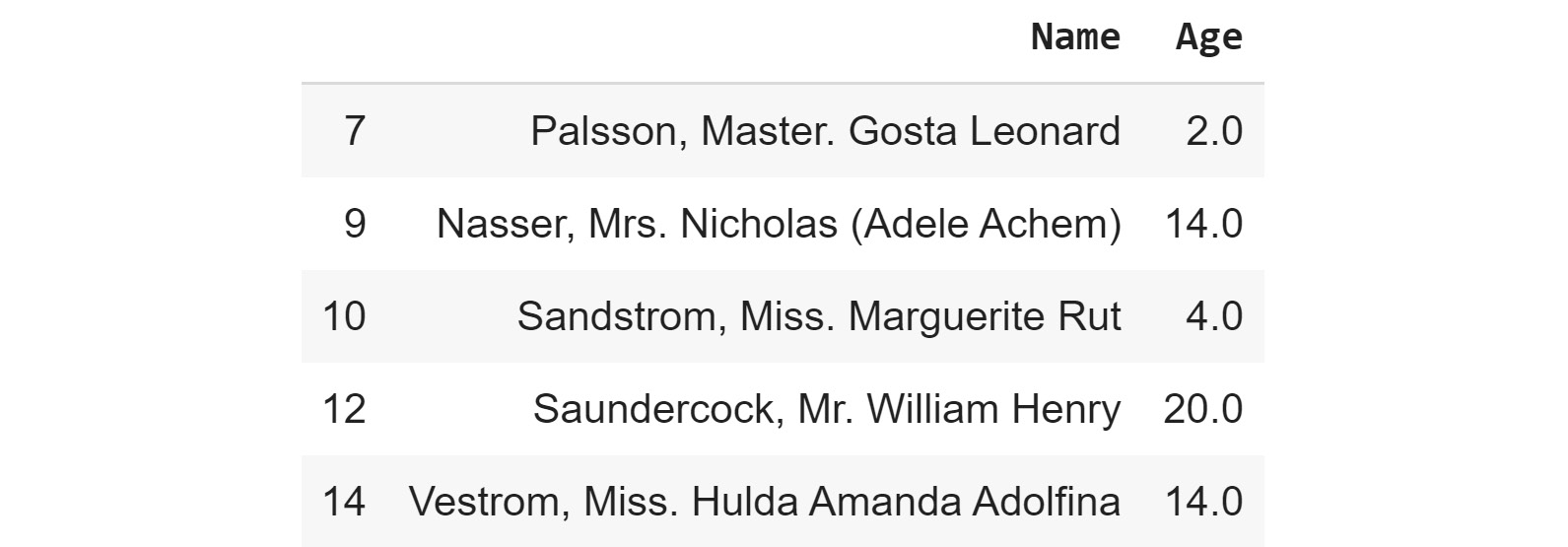Figure 1.10: List of passengers' names and ages for those passengers under the age of 21

2. Count how many child passengers there were, as shown here:
`print(len(child_passengers))`

The output will be as follows:

`249`
3. Count how many passengers were between the ages of 21 and 30. Do not use Python's `and` logical operator for this step, but rather the ampersand symbol (`&`). Do this as follows:

Note

The code snippet shown here uses a backslash ( `\` ) to split the logic across multiple lines. When the code is executed, Python will ignore the backslash, and treat the code on the next line as a direct continuation of the current line.

```young_adult_passengers = df.loc[(df.Age > 21) \
& (df.Age < 30)]

The output will be as follows:

`279`
4. Find the passengers who were either first- or third-class ticket holders. Again, we will not use the Python logical `or` operator but the pipe symbol (`|`) instead. Do this as follows:
`df.loc[(df.Pclass == 3) | (df.Pclass ==1)]`

The output will be as follows: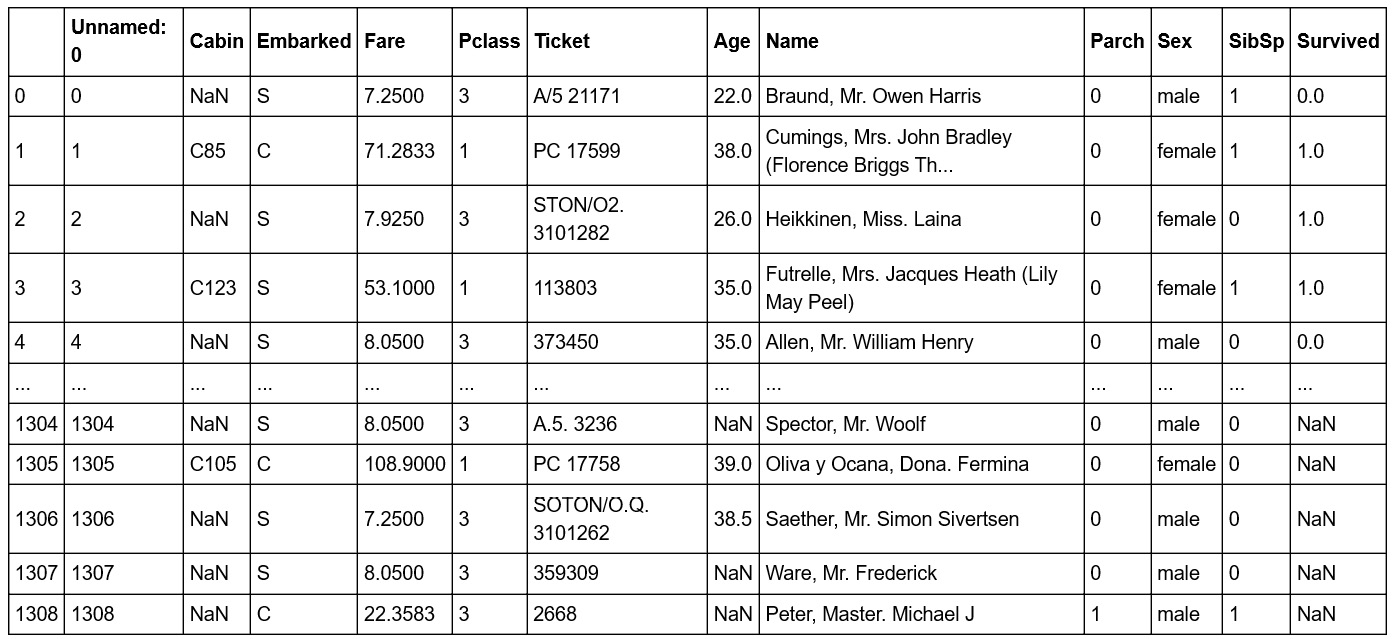Figure 1.11: The number of passengers who were either first- or third-class ticket holders

5. Find the passengers who were not holders of either first- or third-class tickets. Do not simply select those second-class ticket holders, but use the `~` symbol for the `not` logical operator instead. Do this as follows:
`df.loc[~((df.Pclass == 3) | (df.Pclass == 1))]`

The output will be as follows: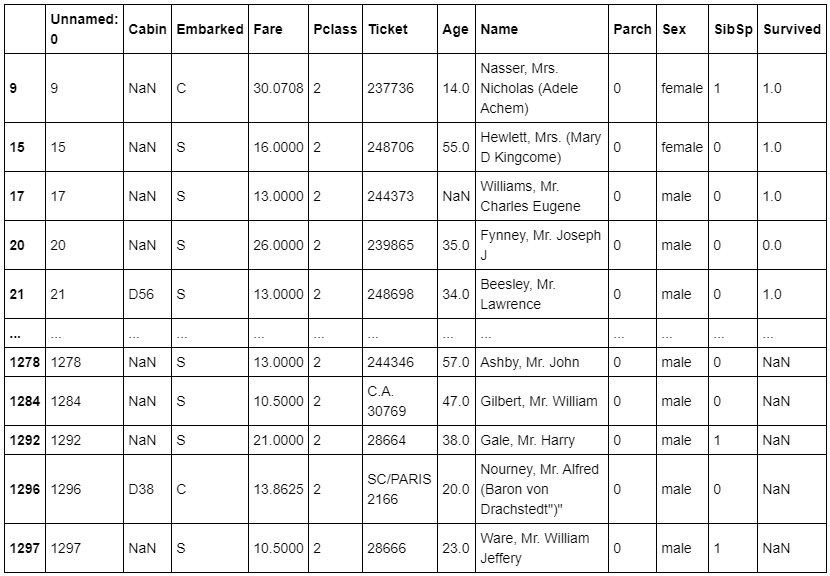Figure 1.12: Count of passengers who were not holders of either first- or third-class tickets

6. We no longer need the `Unnamed: 0` column, so delete it using the `del` operator:
```del df['Unnamed: 0']

The output will be as follows: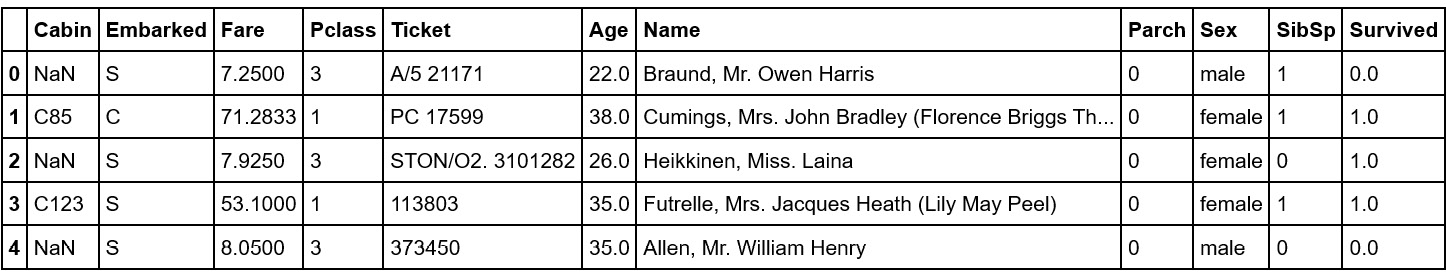Figure 1.13: The del operator

Note

To access the source code for this specific section, please refer to https://packt.live/3empSRO.

You can also run this example online at https://packt.live/3fEsPgK. You must execute the entire Notebook in order to get the desired result.

In this exercise, we have seen how to select data from a `DataFrame` using conditional operators that inspect the data and return the subsets we want. We also saw how to remove a column we didn't need (in this case, the `Unnamed` column simply contained row numbers that are not relevant to analysis). Now, we'll dig deeper into some of the power of pandas.

## Pandas Methods

Now that we are confident with some `pandas` basics, as well as some more advanced indexing and selecting tools, let's look at some other `DataFrame` methods. For a complete list of all methods available in a DataFrame, we can refer to the class documentation.

Note

The pandas documentation is available at https://pandas.pydata.org/pandas-docs/stable/reference/frame.html.

You should now know how many methods are available within a `DataFrame`. There are far too many to cover in detail in this chapter, so we will select a few that will give you a great start in supervised machine learning.

We have already seen the use of one method, `head()`, which provides the first five lines of the DataFrame. We can select more or fewer lines if we wish by providing the number of lines as an argument, as shown here:

```df.head(n=20) # 20 lines

Alternatively, you can use the `tail()` function to see a specified number of lines at the end of the DataFrame.

Another useful method is `describe`, which is a super-quick way of getting the descriptive statistics of the data within a DataFrame. We can see next that the sample size (count), mean, minimum, maximum, standard deviation, and the 25th, 50th, and 75th percentiles are returned for all columns of numerical data in the DataFrame (note that text columns have been omitted):

`df.describe()`

The output will be as follows: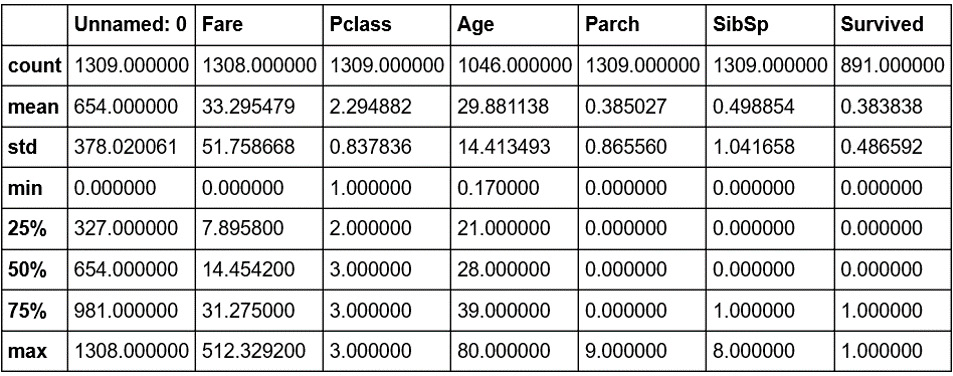Figure 1.14: The describe method

Note that only columns of numerical data have been included within the summary. This simple command provides us with a lot of useful information; looking at the values for `count` (which counts the number of valid samples), we can see that there are 1,046 valid samples in the `Age` category, but 1,308 in `Fare`, and only 891 in `Survived`. We can see that the youngest person was 0.17 years, the average age is 29.898, and the eldest passenger was 80. The minimum fare was £0, with £33.30 the average and £512.33 the most expensive. If we look at the `Survived` column, we have 891 valid samples, with a mean of 0.38, which means about 38% survived.

We can also get these values separately for each of the columns by calling the respective methods of the DataFrame, as shown here:

`df.count()`

The output will be as follows:

```Cabin        295
Embarked    1307
Fare        1308
Pclass      1309
Ticket      1309
Age         1046
Name        1309
Parch       1309
Sex         1309
SibSp       1309
Survived     891
dtype: int64```

But we have some columns that contain text data, such as `Embarked`, `Ticket`, `Name`, and `Sex`. So what about these? How can we get some descriptive information for these columns? We can still use `describe`; we just need to pass it some more information. By default, `describe` will only include numerical columns and will compute the 25th, 50th, and 75th percentiles, but we can configure this to include text-based columns by passing the `include = 'all'` argument, as shown here:

`df.describe(include='all')`

The output will be as follows: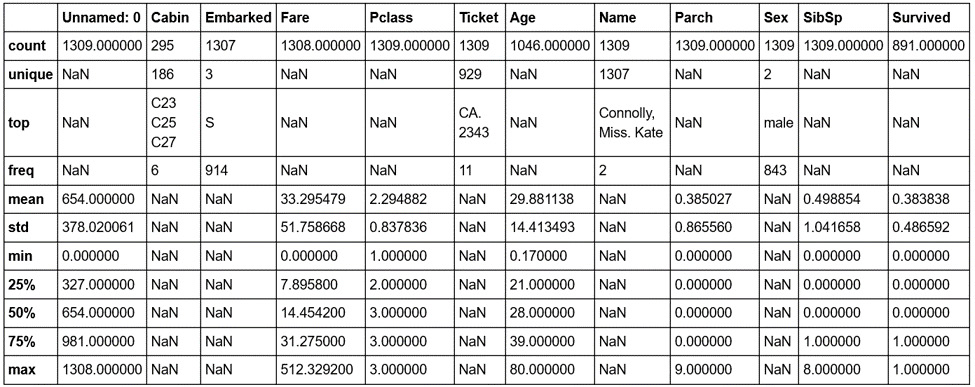Figure 1.15: The describe method with text-based columns

That's better—now we have much more information. Looking at the `Cabin` column, we can see that there are 295 entries, with 186 unique values. The most common values are `C32`, `C25`, and `C27`, and they occur 6 times (from the `freq` value). Similarly, if we look at the `Embarked` column, we see that there are 1,307 entries, 3 unique values, and that the most commonly occurring value is `S`, with 914 entries.

Notice the occurrence of `NaN` values in our `describe` output table. `NaN`, or Not a Number, values are very important within DataFrames as they represent missing or not available data. The ability of the pandas library to read from data sources that contain missing or incomplete information is both a blessing and a curse. Many other libraries would simply fail to import or read the data file in the event of missing information, while the fact that it can be read also means that the missing data must be handled appropriately.

When looking at the output of the `describe` method, you should notice that the Jupyter notebook renders it in the same way as the original DataFrame that we read in using `read_csv`. There is a very good reason for this, as the results returned by the `describe` method are themselves a pandas DataFrame and thus possess the same methods and characteristics as the data read in from the CSV file. This can be easily verified using Python's built-in `type` function, as in the following code:

`type(df.describe(include='all'))`

The output will be as follows:

`pandas.core.frame.DataFrame`

Now that we have a summary of the dataset, let's dive in with a little more detail to get a better understanding of the available data.

Note

A comprehensive understanding of the available data is critical in any supervised learning problem. The source and type of the data, the means by which it is collected, and any errors potentially resulting from the collection process all have an effect on the performance of the final model.

Hopefully, by now, you are comfortable with using pandas to provide a high-level overview of the data. We will now spend some time looking into the data in greater detail.

## Exercise 1.04: Using the Aggregate Method

We have already seen how we can index or select rows or columns from a DataFrame and use advanced indexing techniques to filter the available data based on specific criteria. Another handy method that allows for such selection is the `groupby` method, which provides a quick method for selecting groups of data at a time and provides additional functionality through the `DataFrameGroupBy` object. This exercise is a continuation of Exercise 1.01, Loading and Summarizing the Titanic Dataset:

1. Use the `groupby` method to group the data under the `Embarked` column to find out how many different values for `Embarked` there are:
```embarked_grouped = df.groupby('Embarked')
print(f'There are {len(embarked_grouped)} Embarked groups')```

The output will be as follows:

`There are 3 Embarked groups`
2. Display the output of `embarked_grouped.groups` to find what the `groupby` method actually does:
`embarked_grouped.groups`

The output will be as follows: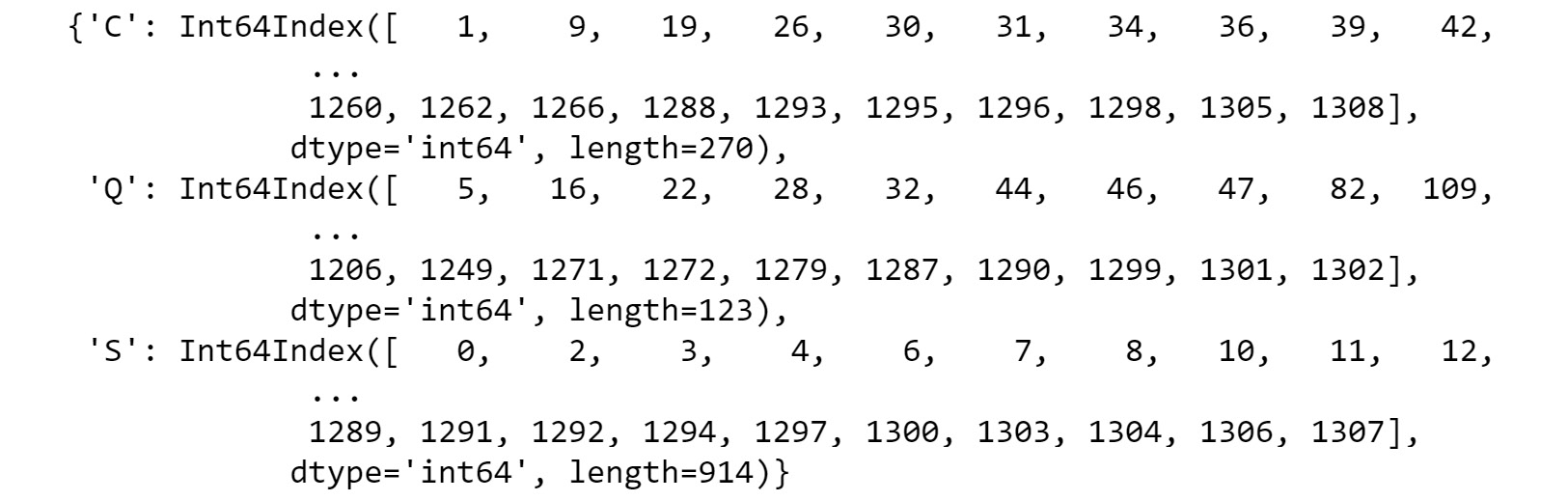Figure 1.16: Output of embarked_grouped.groups

We can see here that the three groups are `C`, `Q`, and `S`, and that `embarked_grouped.groups` is actually a dictionary where the keys are the groups. The values are the rows or indexes of the entries that belong to that group.

3. Use the `iloc` method to inspect row `1` and confirm that it belongs to embarked group `C`:
`df.iloc`

The output will be as follows: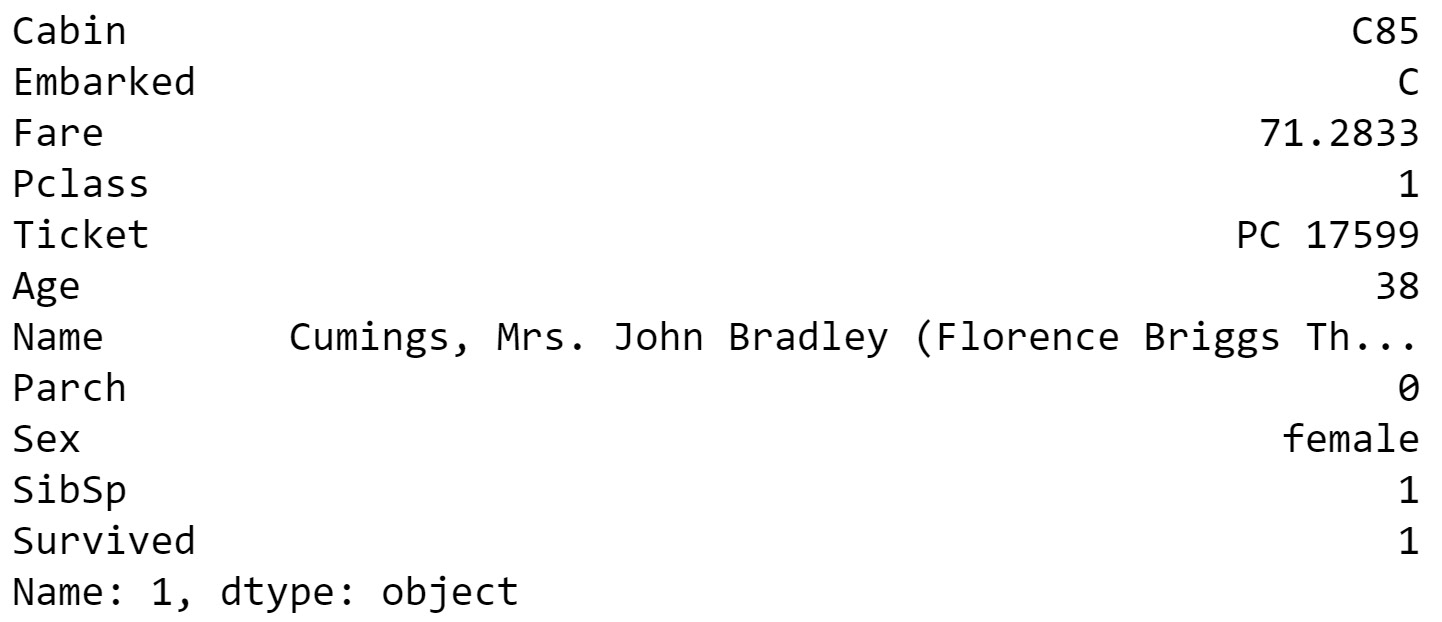Figure 1.17: Inspecting row 1

4. As the groups are a dictionary, we can iterate through them and execute computations on the individual groups. Compute the mean age for each group, as shown here:
```for name, group in embarked_grouped:
print(name, group.Age.mean())```

The output will be as follows:

```C 32.33216981132075
Q 28.63
S 29.245204603580564```
5. Another option is to use the `aggregate` method, or `agg` for short, and provide it with the function to apply across the columns. Use the `agg` method to determine the mean of each group:
`embarked_grouped.agg(np.mean)`

The output will be as follows: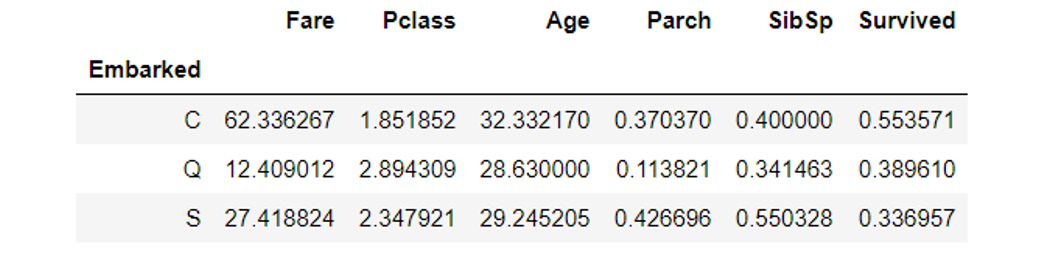Figure 1.18: Using the agg method

So, how exactly does `agg` work and what type of functions can we pass it? Before we can answer these questions, we need to first consider the data type of each column in the DataFrame, as each column is passed through this function to produce the result we see here. Each DataFrame comprises a collection of columns of pandas series data, which, in many ways, operates just like a list. As such, any function that can take a list or a similar iterable and compute a single value as a result can be used with `agg`.

6. Define a simple function that returns the first value in the column and then pass that function through to `agg`, as an example:
```def first_val(x):
return x.values
embarked_grouped.agg(first_val)```

The output will be as follows: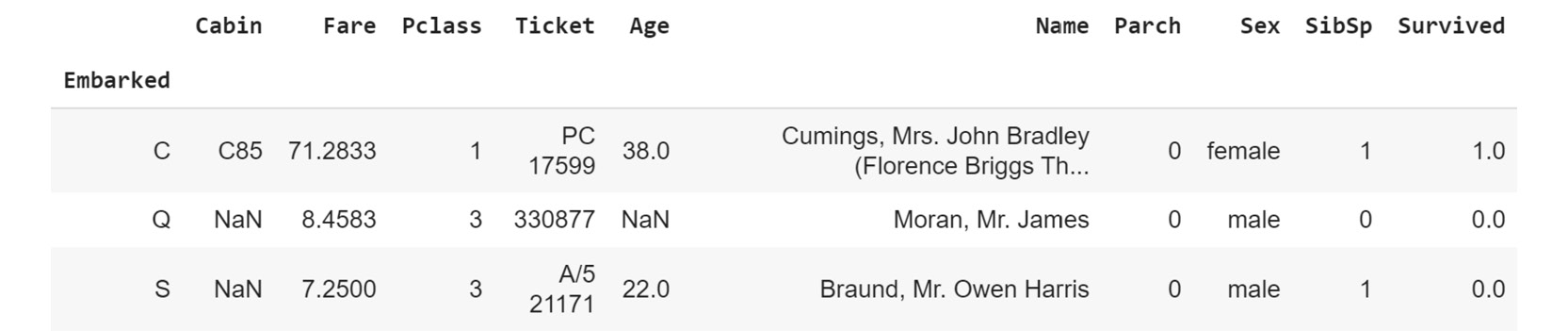Figure 1.19: Using the .agg method with a function

Note

To access the source code for this specific section, please refer to https://packt.live/2NlEkgM.

You can also run this example online at https://packt.live/2AZnq51. You must execute the entire Notebook in order to get the desired result.

In this exercise, we have seen how to group data within a DataFrame, which then allows additional functions to be applied using `.agg()`, such as to calculate group means. These sorts of operations are extremely common in analyzing and preparing data for analysis.

## Quantiles

The previous exercise demonstrated how to find the mean. In statistical data analysis, we are also often interested in knowing the value in a dataset below or above which a certain fraction of the points lie. Such points are called quantiles. For example, if we had a sequence of numbers from 1 to 10,001, the quantile for 25% is the value 2,501. That is, at the value 2,501, 25% of our data lies below that cutoff. Quantiles are often used in data visualizations because they convey a sense of the distribution of the data. In particular, the standard boxplot in Matplotlib draws a box bounded by the first and third of 4 quantiles.

For example, let's establish the 25% quantile of the following dataframe:

```import pandas as pd
df = pd.DataFrame({"A":[1, 6, 9, 9]})
#calculate the 25% quantile over the dataframe
df.quantile(0.25, axis = 0)```

The output will be as follows:

```A    4.75
Name: 0.25, dtype: float64```

As you can see from the preceding output, `4.75` is the `25%` quantile value for the DataFrame.

Note

Later in this book, we'll use the idea of quantiles as we explore the data.

## Lambda Functions

One common and useful way of implementing `agg` is through the use of Lambda functions.

Lambda, or anonymous, functions (also known as inline functions in other languages) are small, single-expression functions that can be declared and used without the need for a formal function definition via the use of the `def` keyword. Lambda functions are essentially provided for convenience and aren't intended to be used for extensive periods. The main benefit of Lambda functions is that they can be used in places where a function might not be appropriate or convenient, such as inside other expressions or function calls. The standard syntax for a Lambda function is as follows (always starting with the `lambda` keyword):

`lambda <input values>: <computation for values to be returned>`

Let's now do an exercise and create some interesting Lambda functions.

## Exercise 1.05: Creating Lambda Functions

In this exercise, we will create a Lambda function that returns the first value in a column and use it with `agg`. This exercise is a continuation of Exercise 1.01, Loading and Summarizing the Titanic Dataset:

1. Write the `first_val` function as a Lambda function, passed to `agg`:
```embarked_grouped = df.groupby('Embarked')
embarked_grouped.agg(lambda x: x.values)```

The output will be as follows: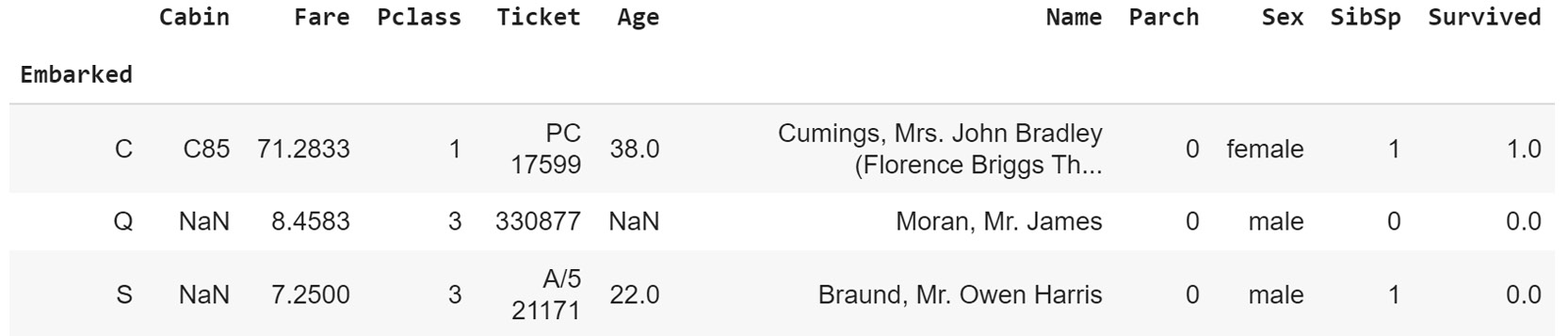Figure 1.20: Using the agg method with a Lambda function

Obviously, we get the same result, but notice how much more convenient the Lambda function was to use, especially given the fact that it is only intended to be used briefly.

2. We can also pass multiple functions to `agg` via a list to apply the functions across the dataset. Pass the Lambda function as well as the NumPy mean and standard deviation functions, like this:
`embarked_grouped.agg([lambda x: x.values, np.mean, np.std])`

The output will be as follows: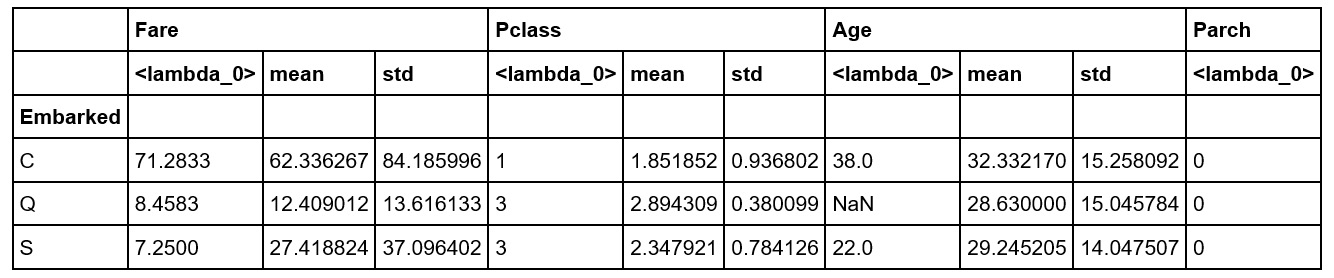Figure 1.21: Using the agg method with multiple Lambda functions

3. Apply `numpy.sum` to the `Fare` column and the Lambda function to the `Age` column by passing `agg` a dictionary where the keys are the columns to apply the function to, and the values are the functions themselves to be able to apply different functions to different columns in the DataFrame:
```embarked_grouped.agg({'Fare': np.sum, \
'Age': lambda x: x.values})```

The output will be as follows:Figure 1.22: Using the agg method with a dictionary of different columns

4. Finally, execute the `groupby` method using more than one column. Provide the method with a list of the columns (`Sex` and `Embarked`) to `groupby`, like this:
```age_embarked_grouped = df.groupby(['Sex', 'Embarked'])
age_embarked_grouped.groups```

The output will be as follows: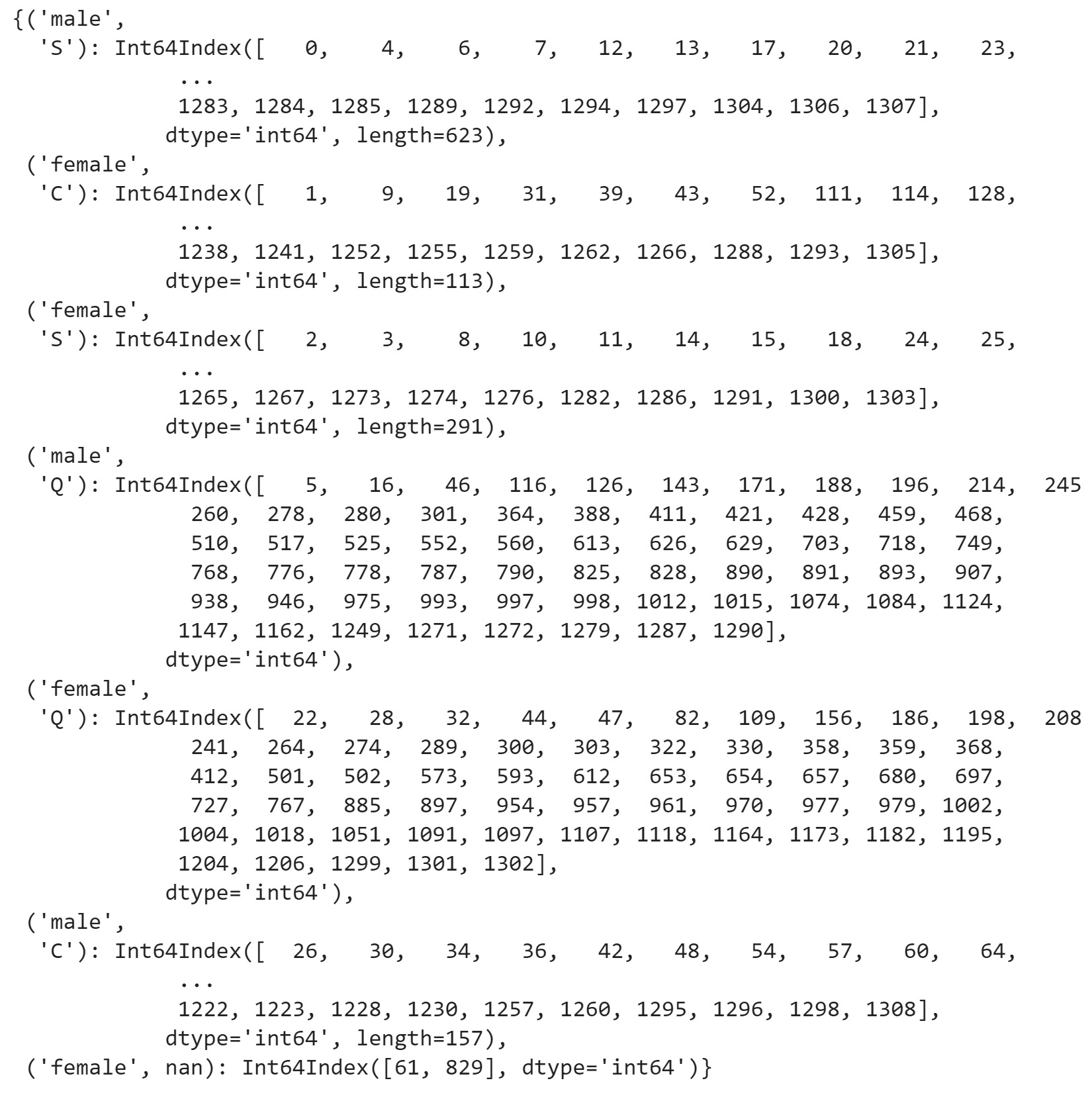Figure 1.23: Using the groupby method with more than one column

Similar to when the groupings were computed by just the `Embarked` column, we can see here that a dictionary is returned where the keys are the combination of the `Sex` and `Embarked` columns returned as a tuple. The first key-value pair in the dictionary is a tuple, `('Male', 'S')`, and the values correspond to the indices of rows with that specific combination. There will be a key-value pair for each combination of unique values in the `Sex` and `Embarked` columns.

Note

To access the source code for this specific section, please refer to https://packt.live/2B1jAZl.

You can also run this example online at https://packt.live/3emqwPe. You must execute the entire Notebook in order to get the desired result.

This concludes our brief exploration of data inspection and manipulation. We now move on to one of the most important topics in data science, data quality.

# Data Quality Considerations

The quality of data used in any machine learning problem, supervised or unsupervised, is critical to the performance of the final model, and should be at the forefront when planning any machine learning project. As a simple rule of thumb, if you have clean data, in sufficient quantity, with a good correlation between the input data type and the desired output, then the specifics regarding the type and details of the selected supervised learning model become significantly less important in achieving a good result.

In reality, however, this is rarely the case. There are usually some issues regarding the quantity of available data, the quality or signal-to-noise ratio in the data, the correlation between the input and output, or some combination of all three factors. As such, we will use this last section of this chapter to consider some of the data quality problems that may occur and some mechanisms for addressing them. Previously, we mentioned that in any machine learning problem, having a thorough understanding of the dataset is critical if we are to construct a high-performing model.

This is particularly the case when looking into data quality and attempting to address some of the issues present within the data. Without a comprehensive understanding of the dataset, additional noise or other unintended issues may be introduced during the data cleaning process, leading to further degradation of performance.

Note

A detailed description of the Titanic dataset and the type of data included is contained in the Loading Data in pandas section. If you need a quick refresher, go back and review that section now.

## Managing Missing Data

As we discussed earlier, the ability of pandas to read data with missing values is both a blessing and a curse and, arguably, is the most common issue that needs to be managed before we can continue with developing our supervised learning model. The simplest, but not necessarily the most effective, method is to just remove or ignore those entries that are missing data. We can easily do this in pandas using the `dropna` method on the DataFrame:

`complete_data = df.dropna()`

There is one very significant consequence of simply dropping rows with missing data and that is we may be throwing away a lot of important information. This is highlighted very clearly in the Titanic dataset as a lot of rows contain missing data. If we were to simply ignore these rows, we would start with a sample size of 1,309 and end with a sample of 183 entries. Developing a reasonable supervised learning model with a little over 10% of the data would be very difficult indeed. The following code displays the use of the `dropna()` method to handle the missing entries:

`len(df)`

The preceding input produces the following output:

`1309`

The `dropna()` method is implemented as follows:

`len(df.dropna())`

The preceding input produces the following output:

`183`

So, with the exception of the early, explorative phase, it is rarely acceptable to simply discard all rows with invalid information. We can identify which rows are actually missing information and whether the missing information is a problem unique to certain columns or is consistent throughout all columns of the dataset. We can use `aggregate` to help us here as well:

`df.aggregate(lambda x: x.isna().sum())`

The output will be as follows:

```Cabin       1014
Embarked       2
Fare           1
Pclass         0
Ticket         0
Age          263
Name           0
Parch          0
Sex            0
SibSp          0
Survived     418
dtype: int64```

Now, this is useful! We can see that the vast majority of missing information is in the `Cabin` column, some in `Age`, and a little more in `Survived`. This is one of the first times in the data cleaning process that we may need to make an educated judgment call.

What do we want to do with the `Cabin` column? There is so much missing information here that, in fact, it may not be possible to use it in any reasonable way. We could attempt to recover the information by looking at the names, ages, and number of parents/siblings and see whether we can match some families together to provide information, but there would be a lot of uncertainty in this process. We could also simplify the column by using the level of the cabin on the ship rather than the exact cabin number, which may then correlate better with name, age, and social status. This is unfortunate as there could be a good correlation between `Cabin` and `Survived`, as perhaps those passengers in the lower decks of the ship may have had a harder time evacuating. We could examine only the rows with valid `Cabin` values to see whether there is any predictive power in the `Cabin` entry; but, for now, we will simply disregard `Cabin` as a reasonable input (or feature).

We can see that the `Embarked` and `Fare` columns only have three missing samples between them. If we decided that we needed the `Embarked` and `Fare` columns for our model, it would be a reasonable argument to simply drop these rows. We can do this using our indexing techniques, where `~` represents the `not` operation, or flipping the result (that is, where `df.Embarked` is not `NaN` and `df.Fare` is not `NaN`):

`df_valid = df.loc[(~df.Embarked.isna()) & (~df.Fare.isna())]`

The missing age values are a little more interesting, as there are too many rows with missing age values to just discard them. But we also have a few more options here, as we can have a little more confidence in some plausible values to fill in. The simplest option would be to simply fill in the missing age values with the mean age for the dataset:

```df_valid[['Age']] = df_valid[['Age']]\
.fillna(df_valid.Age.mean())```

This is okay, but there are probably better ways of filling in the data rather than just giving all 263 people the same value. Remember, we are trying to clean up the data with the goal of maximizing the predictive power of the input features and the survival rate. Giving everyone the same value, while simple, doesn't seem too reasonable. What if we were to look at the average ages of the members of each of the classes (`Pclass`)? This may give a better estimate, as the average age reduces from class 1 through 3, as you can see in the following code:

`df_valid.loc[df.Pclass == 1, 'Age'].mean()`

The preceding input produces the following output:

`37.956806510096975`

Average age for class 2 is as follows:

`df_valid.loc[df.Pclass == 2, 'Age'].mean()`

The preceding input produces the following output:

`29.52440879717283`

Average age for class 3 is as follows:

`df_valid.loc[df.Pclass == 3, 'Age'].mean()`

The preceding input produces the following output:

`26.23396338788047`

What if we were to consider the sex of the person as well as ticket class (social status)? Do the average ages differ here too? Let's find out:

```for name, grp in df_valid.groupby(['Pclass', 'Sex']):
print('%i' % name, name, '%0.2f' % grp['Age'].mean())```

The output will be as follows:

```1 female 36.84
1 male 41.03
2 female 27.50
2 male 30.82
3 female 22.19
3 male 25.86```

We can see here that males in all ticket classes are typically older. This combination of sex and ticket class provides much more resolution than simply filling in all missing fields with the mean age. To do this, we will use the `transform` method, which applies a function to the contents of a series or DataFrame and returns another series or DataFrame with the transformed values. This is particularly powerful when combined with the `groupby` method:

```mean_ages = df_valid.groupby(['Pclass', 'Sex'])['Age'].\
transform(lambda x: \
x.fillna(x.mean()))
df_valid.loc[:, 'Age'] = mean_ages```

There is a lot in these two lines of code, so let's break them down into components. Let's look at the first line:

```mean_ages = df_valid.groupby(['Pclass', 'Sex'])['Age'].\
transform(lambda x: \
x.fillna(x.mean()))```

We are already familiar with `df_valid.groupby(['Pclass', 'Sex'])['Age']`, which groups the data by ticket class and sex and returns only the `Age` column. The `lambda x: x.fillna(x.mean())` Lambda function takes the input pandas series and fills the `NaN` values with the mean value of the series.

The second line assigns the filled values within `mean_ages` to the `Age` column. Note the use of the `loc[:, 'Age']` indexing method, which indicates that all rows within the `Age` column are to be assigned the values contained within `mean_ages`:

`df_valid.loc[:, 'Age'] = mean_ages`

We have described a few different ways of filling in the missing values within the `Age` column, but by no means has this been an exhaustive discussion. There are many more methods that we could use to fill the missing data: we could apply random values within one standard deviation of the mean for the grouped data, and we could also look at grouping the data by sex and the number of parents/children (`Parch`) or by the number of siblings, or by ticket class, sex, and the number of parents/children. What is most important about the decisions made during this process is the end result of the prediction accuracy. We may need to try different options, rerun our models, and consider the effect on the accuracy of final predictions. Thus, selecting the features or components that provide the model with the most predictive power. You will find that, during this process, you will try a few different features, run the model, look at the end result, and repeat this process until you are happy with the performance.

The ultimate goal of this supervised learning problem is to predict the survival of passengers on the Titanic given the information we have available. So, that means that the `Survived` column provides our labels for training. What are we going to do if we are missing 418 of the labels? If this was a project where we had control over the collection of the data and access to its origins, we would obviously correct this by recollecting or asking for the labels to be clarified. With the Titanic dataset, we do not have this ability so we must make another educated judgment call. One approach would be to drop those rows from the training data, and later use a model trained on the (smaller) training set to predict the outcome for the others (this is, in fact, the task given in the Kaggle Titanic competition). In some business problems, we may not have the option of simply ignoring these rows; we might be trying to predict future outcomes of a very critical process and this data is all we have. We could try some unsupervised learning techniques to see whether there are some patterns in the survival information that we could use. However, by estimating the ground truth labels by means of unsupervised techniques, we may introduce significant noise into the dataset, reducing our ability to accurately predict survival.

## Class Imbalance

Missing data is not the only problem that may be present within a dataset. Class imbalance – that is, having more of one class or classes compared to another – can be a significant problem, particularly in the case of classification problems (we'll see more on classification in Chapter 5, Classification Techniques), where we are trying to predict which class (or classes) a sample is from. Looking at our `Survived` column, we can see that there are far more people who perished (`Survived` equals `0`) than survived (`Survived` equals `1`) in the dataset, as you can see in the following code:

`len(df.loc[df.Survived ==1])`

The output is as follows:

`342`

The number of people who perished are:

`len(df.loc[df.Survived ==0])`

The output is as follows:

`549`

If we don't take this class imbalance into account, the predictive power of our model could be significantly reduced as, during training, the model would simply need to guess that the person did not survive to be correct 61% (549 / (549 + 342)) of the time. If, in reality, the actual survival rate was, say, 50%, then when being applied to unseen data, our model would predict did not survive too often.

There are a few options available for managing class imbalance, one of which, similar to the missing data scenario, is to randomly remove samples from the over-represented class until balance has been achieved. Again, this option is not ideal, or perhaps even appropriate, as it involves ignoring available data. A more constructive example may be to oversample the under-represented class by randomly copying samples from the under-represented class in the dataset to boost the number of samples. While removing data can lead to accuracy issues due to discarding useful information, oversampling the under-represented class can lead to being unable to predict the label of unseen data, also known as overfitting (which we will cover in Chapter 6, Ensemble Modeling).

Adding some random noise to the input features for oversampled data may prevent some degree of overfitting, but this is highly dependent on the dataset itself. As with missing data, it is important to check the effect of any class imbalance corrections on the overall model performance. It is relatively straightforward to copy more data into a DataFrame using the `append` method, which works in a very similar fashion to lists. If we wanted to copy the first row to the end of the DataFrame, we would do this:

`df_oversample = df.append(df.iloc)`

## Low Sample Size

The field of machine learning can be considered a branch of the larger field of statistics. As such, the principles of confidence and sample size can also be applied to understand the issues with a small dataset. Recall that if we were to take measurements from a data source with high variance, then the degree of uncertainty in the measurements would also be high and more samples would be required to achieve a specified confidence in the value of the mean. The sample principles can be applied to machine learning datasets. Those datasets with a variance in the features with the most predictive power generally require more samples for reasonable performance as more confidence is also required.

There are a few techniques that can be used to compensate for a reduced sample size, such as transfer learning. However, these lie outside the scope of this book. Ultimately, though, there is only so much that can be done with a small dataset, and significant performance increases may only occur once the sample size is increased.

## Activity 1.01: Implementing Pandas Functions

In this activity, we will test ourselves on the various pandas functions we have learned about in this chapter. We will use the same Titanic dataset for this.

The steps to be performed are as follows:

1. Open a new Jupyter notebook.
2. Use pandas to load the Titanic dataset and use the `head` function on the dataset to display the top rows of the dataset. Describe the summary data for all columns.
3. We don't need the `Unnamed: 0` column. In Exercise 1.03: Advanced Indexing and Selection, we demonstrated how to remove the column using the `del` command. How else could we remove this column? Remove this column without using `del`.
4. Compute the mean, standard deviation, minimum, and maximum values for the columns of the DataFrame without using `describe`. Note that you can find the minimum and maximum values using the `df.min()` and `df.max()` functions.
5. Use the `quantile` method to get values for the 33, 66, and 99% quantiles.
6. Find how many passengers were from each class using the `groupby` method.
7. Find how many passengers were from each class answer by using selecting/indexing methods to count the members of each class. You can use the `unique()` method to find out the unique values of each class.

Confirm that the answers to Step 6 and Step 7 match.

8. Determine who the eldest passenger in third class was.
9. For a number of machine learning problems, it is very common to scale the numerical values between 0 and 1. Use the `agg` method with Lambda functions to scale the `Fare` and `Age` columns between 0 and 1.
10. There is one individual in the dataset without a listed `Fare` value, which can be established as follows:
```df_nan_fare = df.loc[(df.Fare.isna())]
df_nan_fare```

The output will be as follows: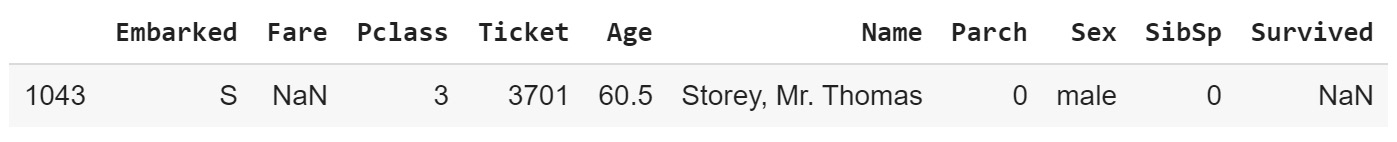Figure 1.24: Individual without a listed fare value

11. Replace the `NaN` value of this row in the main DataFrame with the mean `Fare` value for those corresponding to the same class and `Embarked` location using the `groupby` method.

The output will be as follows: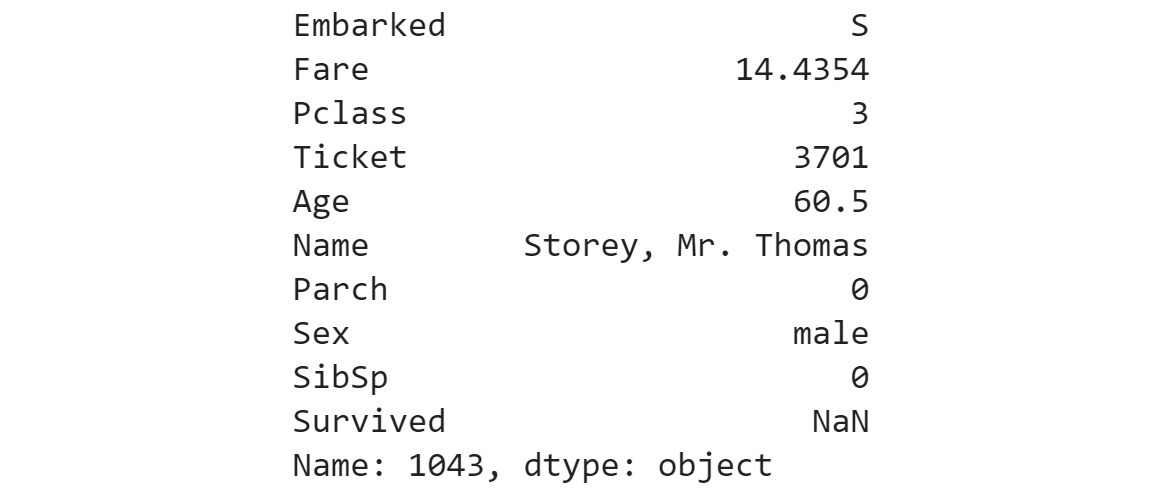Figure 1.25: Output for the individual without listed fare details

Note

The solution for this activity can be found via this link.

With this activity, we have reviewed all the basic data loading, inspection, and manipulation methods, as well as some basic summary statistics methods.

# Summary

In this chapter, we introduced the concept of supervised machine learning, along with a number of use cases, including the automation of manual tasks such as identifying hairstyles from the 1960s and 1980s. In this introduction, we encountered the concept of labeled datasets and the process of mapping one information set (the input data or features) to the corresponding labels. We took a practical approach to the process of loading and cleaning data using Jupyter notebooks and the extremely powerful pandas library. Note that this chapter has only covered a small fraction of the functionality within pandas, and that an entire book could be dedicated to the library itself. It is recommended that you become familiar with reading the pandas documentation and continue to develop your pandas skills through practice. The final section of this chapter covered a number of data quality issues that need to be considered to develop a high-performing supervised learning model, including missing data, class imbalance, and low sample sizes. We discussed a number of options for managing such issues and emphasized the importance of checking these mitigations against the performance of the model. In the next chapter, we will extend the data cleaning process that we covered and investigate the data exploration and visualization process. Data exploration is a critical aspect of any machine learning solution since without a comprehensive knowledge of the dataset, it would be almost impossible to model the information provided.

• Blaine Bateman

Blaine Bateman has more than 35 years of experience working with various industries from government R&D to startups to \$1B public companies. His experience focuses on analytics including machine learning and forecasting. His hands-on abilities include Python and R coding, Keras/Tensorflow, and AWS & Azure machine learning services. As a machine learning consultant, he has developed and deployed actual ML models in industry.

Browse publications by this author
• Ashish Ranjan Jha

Ashish Ranjan Jha received his bachelor's degree in electrical engineering from IIT Roorkee (India), a master's degree in Computer Science from EPFL (Switzerland), and an MBA degree from Quantic School of Business (Washington). He has received a distinction in all 3 of his degrees. He has worked for large technology companies, including Oracle and Sony as well as the more recent tech unicorns such as Revolut, mostly focused on artificial intelligence. He currently works as a machine learning engineer.

Ashish has worked on a range of products and projects, from developing an app that uses sensor data to predict the mode of transport to detecting fraud in car damage insurance claims. Besides being an author, machine learning engineer, and data scientist, he also blogs frequently on his personal blog site about the latest research and engineering topics around machine learning.

Browse publications by this author
• Benjamin Johnston

Benjamin Johnston is a senior data scientist for one of the world's leading data-driven MedTech companies and is involved in the development of innovative digital solutions throughout the entire product development pathway, from problem definition to solution research and development, through to final deployment. He is currently completing his Ph.D. in machine learning, specializing in image processing and deep convolutional neural networks. He has more than 10 years of experience in medical device design and development, working in a variety of technical roles, and holds first-class honors bachelor's degrees in both engineering and medical science from the University of Sydney, Australia.

Browse publications by this author
• Ishita Mathur

Ishita Mathur has worked as a data scientist for 2.5 years with product-based start-ups working with business concerns in various domains and formulating them as technical problems that can be solved using data and machine learning. Her current work at GO-JEK involves the end-to-end development of machine learning projects, by working as part of a product team on defining, prototyping, and implementing data science models within the product. She completed her masters' degree in high-performance computing with data science at the University of Edinburgh, UK, and her bachelor's degree with honors in physics at St. Stephen's College, Delhi.

Browse publications by this author
Latest Reviews (1 reviews total)